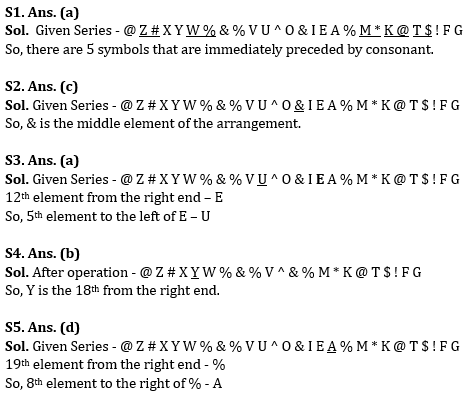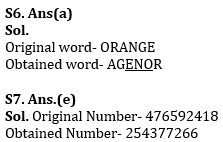Latest Banking jobs   »   IBPS reasoning

# Reasoning Quiz For IBPS PO Prelims 2023-11th September

Directions (1-5): Study the following arrangement of symbols & alphabets carefully & answer the following questions:

@ Z # X Y W % & % V U ^ O & I E A % M * K @ T \$ ! F G

Q1. How many symbols are in the arrangement which are immediately preceded by consonant?
(a) Five
(b) Eight
(c) Nine
(d) Ten
(e) None of the above

Q2. Which of the following is the middle element of the arrangement?
(a) ^
(b) O
(c) &
(d) I
(e) None of the above

Q3. Which of the following is the fifth element to the left of 12th element from right end?
(a) U
(b) V
(c) %
(d) O
(e) None of the above

Q4. If we drop all the vowels from the given arrangement then which of the following element is 18th from the right end?
(a) X
(b) Y
(c) %
(d) Z
(e) None of the above

Q5. Which of the following is the eighth element to the right of 19th element from right end?
(a) %
(b) M
(c) E
(d) A
(e) None of the above

Q6. If all the letters in the word ORANGE are arranged in alphabetical order from left to right in such a way that one vowel is arranged followed by one consonant, then second vowel is arranged followed by second consonants and so on. How many letters will be in between G and R after the arrangement?
(a) Three
(b) One
(c) None
(d) Two
(e) Five

Q7. If in the number 476592418, 5 is added to each of the digit which is less than three and 2 is subtracted from each of the digit which is greater than three then how many digits are repeating in the number thus formed?
(a) One
(b) Two
(c) None
(d) Four
(e) Three

Q8. Which of the following will be definitely true if the given expression W=L≥O=M>U≥C>H≥Q>P is definitely true?
(a) W <U
(b) L ≥ P
(c) P > M
(d) O ≥ H
(e) C < L

Q9. The position of how many alphabets will remain unchanged if each of the alphabets in the word ‘SIGNIFICANT’ is arranged in alphabetical order from left to right?
(a) Two
(b) Three
(c) One
(d) Four
(e) Five

Q10.Which of the following elements should come in a place ‘?’ ?
IR10 KP12 MN14 OL16 ?
(a) RS19
(b) RI19
(c) QR18
(d) QJ18
(e) None of these

SolutionsS8. Ans.(e)

S9. Ans(b)
Sol.
Original word – SIGNIFICANT
Obtained word-ACFGIIINNST

S10.Ans(d)

## FAQs

### When will the IBPS PO prelims 2023 be conducted?

IBPS PO Prelims will be conducted on 23, 30 September, and 1 October 2023.

#### Congratulations!Union Budget 2023-24: Free PDF